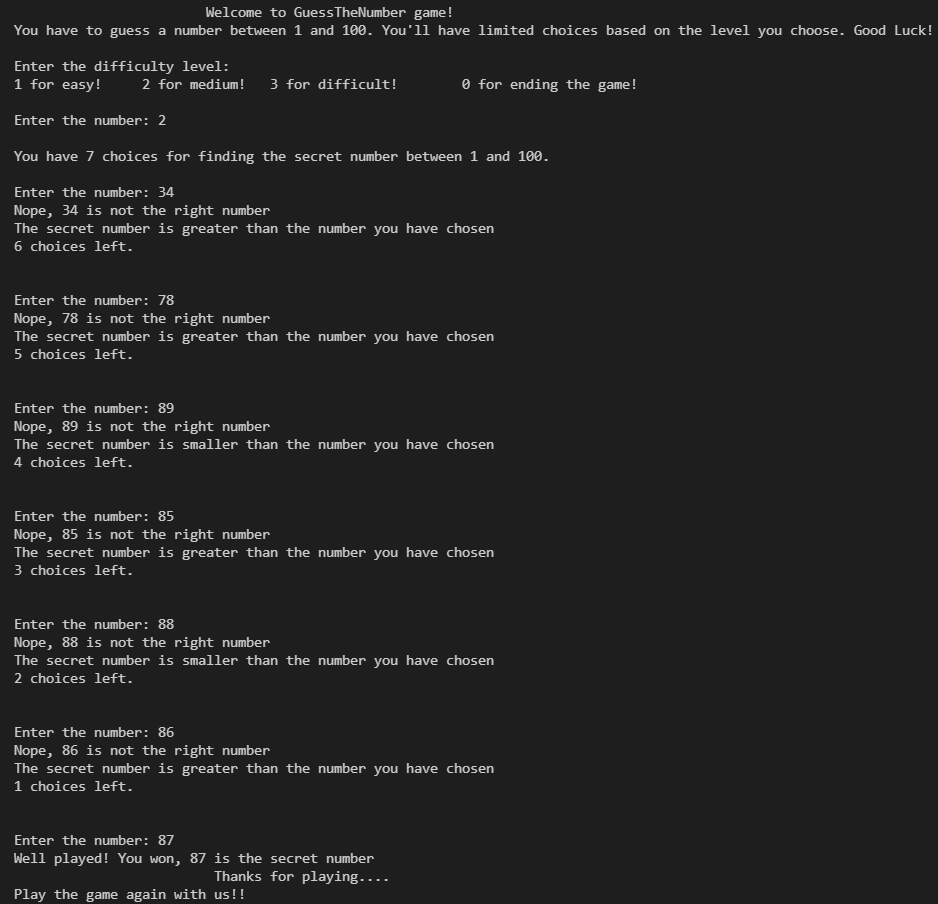Open In App

# Number Guessing Game in C++ using rand() Function

In this article, we will develop a C++ game for guessing a secret number with three difficulty levels.

• In this game, the computer generates a secret number in the range of 1 to 100, and the player has to guess it.
• The game has three difficulty levels. A player’s chances of guessing are limited by the level they choose. The easy level gives the player 10 chances to guess the secret number, the medium level 7 chances, whereas the difficult level only offers 5 chances.
• During the game, a player tells the computer his assumption about a number, and the computer tells if the player is correct. If his number is less or more than the secret number, the computer informs the player, and the player tries again.
• The player can also end the game at any time.

Approach

Step 1: Generate a Random Secret Number Between 1 & 100
No function in C++ generates a random function in a given range. Therefore, we will use the rand() function. We will need the cstdlib library to use rand(). The formula to generate a random function within a range is

`randomNumber = (rand() % (upper-lower) + 1)`

Let’s say we wish to generate a number between 1 and 100, so upper equals 100, and lower equals 1. So, the formula becomes

`randomNumber = (rand() % (100-1) + 1)`

Now, every time we run the program, the computer generates the same random number as the secret number, making it quite repetitive, and boring and this one-time game loses its essence.
To generate different random numbers each time the program runs, we will use the srand(time(0)) function. This function changes the seed each time the program runs. time(0) returns the number of seconds that the system clock shows. Since we will be using the time function, we will have to include the ctime library.

Step 2: Ask the User to Select the Level of Difficulty
While loops let us implement a menu-driven program in which the player can select the degree of difficulty. The user can press 1 to select the easy level, 2 for medium, and 3 for the difficulty level.

Step 3: Determine the Number of Chances the Player has Based on the Level of Difficulty
When the player selects the easy level, he gets 10 chances to guess the secret number. With medium, he has 7 chances while with hard, he has 5.

Step 4: Check Whether the Entered Number is Equal to the Secret Number
Using the if-else construct, we will check if the entered number matches the secret number.

• As the player is granted 10 chances at the easy level, we will iterate from 1 to 10 to determine if the entered number matches the actual secret number. Since the player has only seven choices in level medium, we iterate from 1 to 7 to check if the number matches the secret number. We will iterate from 1 to 5 if the player selects hard level since there are only 5 choices.
• We will display that the secret number is smaller than the chosen number if the entered number is smaller than the secret number.
• Whenever the entered number exceeds the secret number, we will display that the secret number is greater, which serves as a hint for the player.
• We will also display the number of choices left.
• Once the number entered matches the secret number, the player will be notified that he has won.
• The game ends if the player cannot guess the number and he or she has no more choices left. This terminates the game.

Implementation:

## C++

 `#include ``#include ``#include ``using` `namespace` `std;` `int` `main()``{` `    ``cout << ``"\n\t\t\tWelcome to GuessTheNumber game!"``         ``<< endl;``    ``cout << ``"You have to guess a number between 1 and 100. "``            ``"You'll have limited choices based on the "``            ``"level you choose. Good Luck!"``         ``<< endl;` `    ``while` `(``true``) {``        ``cout << ``"\nEnter the difficulty level: \n"``;``        ``cout << ``"1 for easy!\t"``;``        ``cout << ``"2 for medium!\t"``;``        ``cout << ``"3 for difficult!\t"``;``        ``cout << ``"0 for ending the game!\n"` `<< endl;` `        ``// select the level of difficulty``        ``int` `difficultyChoice;``        ``cout << ``"Enter the number: "``;``        ``cin >> difficultyChoice;` `        ``// generating the secret number``        ``srand``(``time``(0));``        ``int` `secretNumber = 1 + (``rand``() % 100);``        ``int` `playerChoice;` `        ``// Difficulty Level:Easy``        ``if` `(difficultyChoice == 1) {``            ``cout << ``"\nYou have 10 choices for finding the "``                    ``"secret number between 1 and 100."``;``            ``int` `choicesLeft = 10;``            ``for` `(``int` `i = 1; i <= 10; i++) {` `                ``// prompting the player to guess the secret``                ``// number``                ``cout << ``"\n\nEnter the number: "``;``                ``cin >> playerChoice;` `                ``// determining if the playerChoice matches``                ``// the secret number``                ``if` `(playerChoice == secretNumber) {``                    ``cout << ``"Well played! You won, "``                         ``<< playerChoice``                         ``<< ``" is the secret number"` `<< endl;``                    ``cout << ``"\t\t\t Thanks for playing...."``                         ``<< endl;``                    ``cout << ``"Play the game again with "``                            ``"us!!\n\n"``                         ``<< endl;``                    ``break``;``                ``}``                ``else` `{``                    ``cout << ``"Nope, "` `<< playerChoice``                         ``<< ``" is not the right number\n"``;``                    ``if` `(playerChoice > secretNumber) {``                        ``cout << ``"The secret number is "``                                ``"smaller than the number "``                                ``"you have chosen"``                             ``<< endl;``                    ``}``                    ``else` `{``                        ``cout << ``"The secret number is "``                                ``"greater than the number "``                                ``"you have chosen"``                             ``<< endl;``                    ``}``                    ``choicesLeft--;``                    ``cout << choicesLeft << ``" choices left. "``                         ``<< endl;``                    ``if` `(choicesLeft == 0) {``                        ``cout << ``"You couldn't find the "``                                ``"secret number, it was "``                             ``<< secretNumber``                             ``<< ``", You lose!!\n\n"``;``                        ``cout << ``"Play the game again to "``                                ``"win!!!\n\n"``;``                    ``}``                ``}``            ``}``        ``}` `        ``// Difficulty level : Medium``        ``else` `if` `(difficultyChoice == 2) {``            ``cout << ``"\nYou have 7 choices for finding the "``                    ``"secret number between 1 and 100."``;``            ``int` `choicesLeft = 7;``            ``for` `(``int` `i = 1; i <= 7; i++) {` `                ``// prompting the player to guess the secret``                ``// number``                ``cout << ``"\n\nEnter the number: "``;``                ``cin >> playerChoice;` `                ``// determining if the playerChoice matches``                ``// the secret number``                ``if` `(playerChoice == secretNumber) {``                    ``cout << ``"Well played! You won, "``                         ``<< playerChoice``                         ``<< ``" is the secret number"` `<< endl;``                    ``cout << ``"\t\t\t Thanks for playing...."``                         ``<< endl;``                    ``cout << ``"Play the game again with "``                            ``"us!!\n\n"``                         ``<< endl;``                    ``break``;``                ``}``                ``else` `{``                    ``cout << ``"Nope, "` `<< playerChoice``                         ``<< ``" is not the right number\n"``;``                    ``if` `(playerChoice > secretNumber) {``                        ``cout << ``"The secret number is "``                                ``"smaller than the number "``                                ``"you have chosen"``                             ``<< endl;``                    ``}``                    ``else` `{``                        ``cout << ``"The secret number is "``                                ``"greater than the number "``                                ``"you have chosen"``                             ``<< endl;``                    ``}``                    ``choicesLeft--;``                    ``cout << choicesLeft << ``" choices left. "``                         ``<< endl;``                    ``if` `(choicesLeft == 0) {``                        ``cout << ``"You couldn't find the "``                                ``"secret number, it was "``                             ``<< secretNumber``                             ``<< ``", You lose!!\n\n"``;``                        ``cout << ``"Play the game again to "``                                ``"win!!!\n\n"``;``                    ``}``                ``}``            ``}``        ``}``        ``// Difficulty level : Medium``        ``else` `if` `(difficultyChoice == 3) {``            ``cout << ``"\nYou have 5 choices for finding the "``                    ``"secret number between 1 and 100."``;``            ``int` `choicesLeft = 5;``            ``for` `(``int` `i = 1; i <= 5; i++) {` `                ``// prompting the player to guess the secret``                ``// number``                ``cout << ``"\n\nEnter the number: "``;``                ``cin >> playerChoice;` `                ``// determining if the playerChoice matches``                ``// the secret number``                ``if` `(playerChoice == secretNumber) {``                    ``cout << ``"Well played! You won, "``                         ``<< playerChoice``                         ``<< ``" is the secret number"` `<< endl;``                    ``cout << ``"\t\t\t Thanks for playing...."``                         ``<< endl;``                    ``cout << ``"Play the game again with "``                            ``"us!!\n\n"``                         ``<< endl;``                    ``break``;``                ``}``                ``else` `{``                    ``cout << ``"Nope, "` `<< playerChoice``                         ``<< ``" is not the right number\n"``;``                    ``if` `(playerChoice > secretNumber) {``                        ``cout << ``"The secret number is "``                                ``"smaller than the number "``                                ``"you have chosen"``                             ``<< endl;``                    ``}``                    ``else` `{``                        ``cout << ``"The secret number is "``                                ``"greater than the number "``                                ``"you have chosen"``                             ``<< endl;``                    ``}``                    ``choicesLeft--;``                    ``cout << choicesLeft << ``" choices left. "``                         ``<< endl;``                    ``if` `(choicesLeft == 0) {``                        ``cout << ``"You couldn't find the "``                                ``"secret number, it was "``                             ``<< secretNumber``                             ``<< ``", You lose!!\n\n"``;``                        ``cout << ``"Play the game again to "``                                ``"win!!!\n\n"``;``                    ``}``                ``}``            ``}``        ``}``        ``// To end the game``        ``else` `if` `(difficultyChoice == 0) {``            ``exit``(0);``        ``}``        ``else` `{``            ``cout << ``"Wrong choice, Enter valid choice to "``                    ``"play the game! (0,1,2,3)"``                 ``<< endl;``        ``}``    ``}``    ``return` `0;``}`

Output: Let’s try the game with Medium difficulty, and we’ll see how it goes.Output: Guess the secret NumberOutput

Time complexity: O(1)
Auxiliary Space: O(1)